# Round Robin | Round Robin Scheduling | Examples

## Round Robin Scheduling-

In Round Robin Scheduling,

• CPU is assigned to the process on the basis of FCFS for a fixed amount of time.
• This fixed amount of time is called as time quantum or time slice.
• After the time quantum expires, the running process is preempted and sent to the ready queue.
• Then, the processor is assigned to the next arrived process.
• It is always preemptive in nature.

 Round Robin Scheduling is FCFS Scheduling with preemptive mode.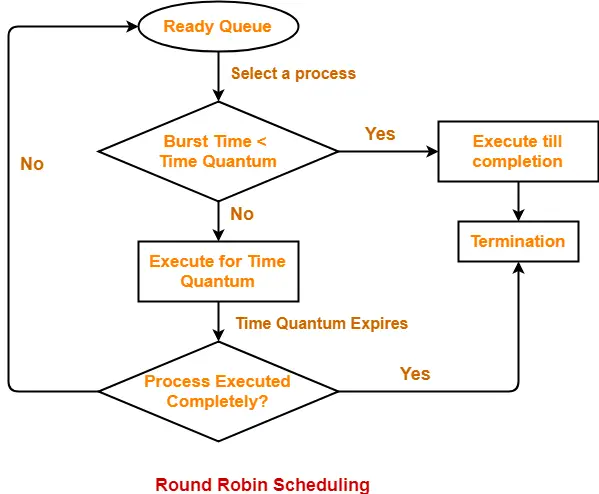• It gives the best performance in terms of average response time.
• It is best suited for time sharing system, client server architecture and interactive system.

• It leads to starvation for processes with larger burst time as they have to repeat the cycle many times.
• Its performance heavily depends on time quantum.
• Priorities can not be set for the processes.

## Important Notes-

### Note-01:

With decreasing value of time quantum,

• Number of context switch increases
• Response time decreases
• Chances of starvation decreases

Thus, smaller value of time quantum is better in terms of response time.

### Note-02:

With increasing value of time quantum,

• Number of context switch decreases
• Response time increases
• Chances of starvation increases

Thus, higher value of time quantum is better in terms of number of context switch.

### Note-03:

• With increasing value of time quantum, Round Robin Scheduling tends to become FCFS Scheduling.
• When time quantum tends to infinity, Round Robin Scheduling becomes FCFS Scheduling.

### Note-04:

• The performance of Round Robin scheduling heavily depends on the value of time quantum.
• The value of time quantum should be such that it is neither too big nor too small.

## Problem-01:

Consider the set of 5 processes whose arrival time and burst time are given below-

 Process Id Arrival time Burst time P1 0 5 P2 1 3 P3 2 1 P4 3 2 P5 4 3

If the CPU scheduling policy is Round Robin with time quantum = 2 unit, calculate the average waiting time and average turn around time.

## Solution-

### Gantt Chart-

P5, P1, P2, P5, P4, P1, P3, P2, P1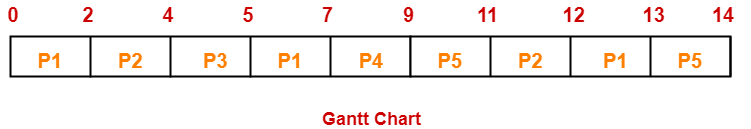Now, we know-

• Turn Around time = Exit time – Arrival time
• Waiting time = Turn Around time – Burst time

Also read- Various Times of Process

 Process Id Exit time Turn Around time Waiting time P1 13 13 – 0 = 13 13 – 5 = 8 P2 12 12 – 1 = 11 11 – 3 = 8 P3 5 5 – 2 = 3 3 – 1 = 2 P4 9 9 – 3 = 6 6 – 2 = 4 P5 14 14 – 4 = 10 10 – 3 = 7

Now,

• Average Turn Around time = (13 + 11 + 3 + 6 + 10) / 5 = 43 / 5 = 8.6 unit
• Average waiting time = (8 + 8 + 2 + 4 + 7) / 5 = 29 / 5 = 5.8 unit

## Problem-02:

Consider the set of 6 processes whose arrival time and burst time are given below-

 Process Id Arrival time Burst time P1 0 4 P2 1 5 P3 2 2 P4 3 1 P5 4 6 P6 6 3

If the CPU scheduling policy is Round Robin with time quantum = 2, calculate the average waiting time and average turn around time.

## Solution-

### Gantt chart-

P5, P6, P2, P5, P6, P2, P5, P4, P1, P3, P2, P1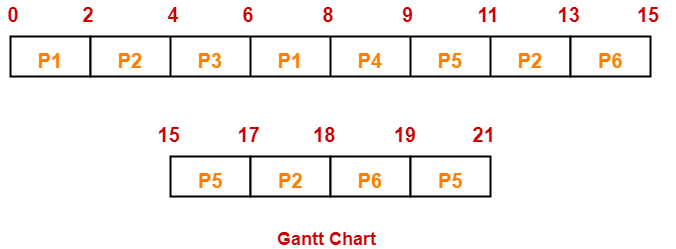Now, we know-

• Turn Around time = Exit time – Arrival time
• Waiting time = Turn Around time – Burst time

 Process Id Exit time Turn Around time Waiting time P1 8 8 – 0 = 8 8 – 4 = 4 P2 18 18 – 1 = 17 17 – 5 = 12 P3 6 6 – 2 = 4 4 – 2 = 2 P4 9 9 – 3 = 6 6 – 1 = 5 P5 21 21 – 4 = 17 17 – 6 = 11 P6 19 19 – 6 = 13 13 – 3 = 10

Now,

• Average Turn Around time = (8 + 17 + 4 + 6 + 17 + 13) / 6 = 65 / 6 = 10.84 unit
• Average waiting time = (4 + 12 + 2 + 5 + 11 + 10) / 6 = 44 / 6 = 7.33 unit

## Problem-03:

Consider the set of 6 processes whose arrival time and burst time are given below-

 Process Id Arrival time Burst time P1 5 5 P2 4 6 P3 3 7 P4 1 9 P5 2 2 P6 6 3

If the CPU scheduling policy is Round Robin with time quantum = 3, calculate the average waiting time and average turn around time.

## Solution-

### Gantt chart-

P3, P1, P4, P2, P3, P6, P1, P4, P2, P3, P5, P4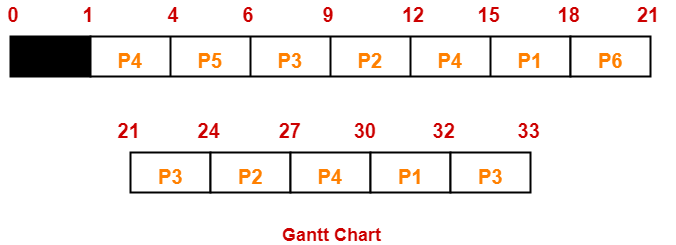Now, we know-

• Turn Around time = Exit time – Arrival time
• Waiting time = Turn Around time – Burst time

 Process Id Exit time Turn Around time Waiting time P1 32 32 – 5 = 27 27 – 5 = 22 P2 27 27 – 4 = 23 23 – 6 = 17 P3 33 33 – 3 = 30 30 – 7 = 23 P4 30 30 – 1 = 29 29 – 9 = 20 P5 6 6 – 2 = 4 4 – 2 = 2 P6 21 21 – 6 = 15 15 – 3 = 12

Now,

• Average Turn Around time = (27 + 23 + 30 + 29 + 4 + 15) / 6 = 128 / 6 = 21.33 unit
• Average waiting time = (22 + 17 + 23 + 20 + 2 + 12) / 6 = 96 / 6 = 16 unit

## Problem-04:

Four jobs to be executed on a single processor system arrive at time 0 in the order A, B, C, D. Their burst CPU time requirements are 4, 1, 8, 1 time units respectively. The completion time of A under round robin scheduling with time slice of one time unit is-

1. 10
2. 4
3. 8
4. 9

## Solution-

 Process Id Arrival time Burst time A 0 4 B 0 1 C 0 8 D 0 1

### Gantt chart-

C, A, C, A, C, A, D, C, B, A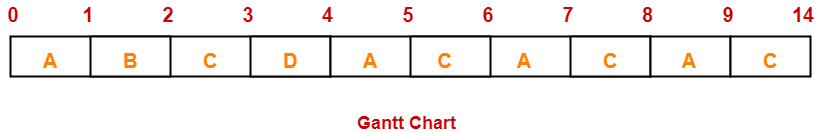Clearly, completion time of process A = 9 unit.

Thus, Option (D) is correct.

To gain better understanding about Round Robin Scheduling,

Watch this Video Lecture

Next Article- Priority Scheduling

Get more notes and other study material of Operating System.

Watch video lectures by visiting our YouTube channel LearnVidFun.

SummaryArticle Name
Round Robin | Round Robin Scheduling | Examples
Description
Round Robin Scheduling is a CPU scheduling algorithm that assigns CPU on basis of FCFS for fixed time called as time quantum. Round Robin Scheduling Example. Round Robin Scheduling is FCFS Scheduling with preemptive mode.
Author
Publisher Name
Gate Vidyalay
Publisher Logo